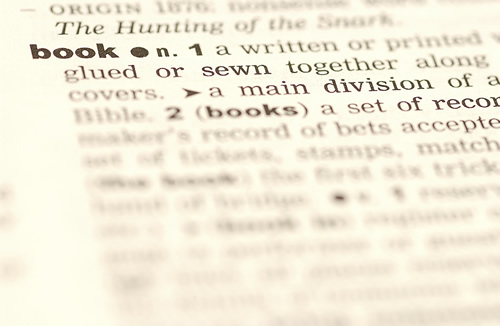### Math Vocabulary WordsMath Vocabulary Words
• sum
• digits
• difference
• fact family
• even odd
• odd number
• comparison symbol (<,>,=)
• expanded form
• expanded notation
• regrouping
• rounding, estimate, about, nearest, around
• perimeter
• right angle
• whole number
• fraction
• numerator
• denominator
• cross multiplication
• factor
• product
• area
• perimeter